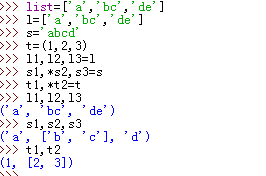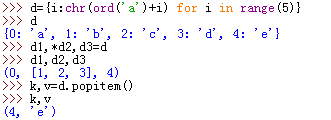Jquery中文网 www.jquerycn.cn
Jquery中文网 >  Python编程  >  Python入门  >  正文 特色的Python序列解包、链式赋值、链式比较

# 特色的Python序列解包、链式赋值、链式比较

jquery中文网为您提供特色的Python序列解包、链式赋值、链式比较等资源，欢迎您收藏本站，我们将为您提供最新的特色的Python序列解包、链式赋值、链式比较资源```\>>>l,s,t,d=['a','bc','de'],'abcd',(1,2,3),{1:'a',2:'b'} #定义4个列表、字符串、元组和字典变量；
\>>>l1,l2,l3=l? #用l1、l2、l3解包列表，l1,l2,l3分别得到值'a', 'bc', 'de'；
\>>>s1,*s2,s3=s #用s1、s2、s3解包字符串，s1,s2,s3分别得到值'a', ['b', 'c'], 'd',注意星号的使用；
\>>>t1,*t2=t #用t1、t2解包元组，t1,t2分别得到值1, [2, 3]；
\>>>d={i:chr(ord('a') i) for i in range(5)}? #d={0: 'a', 1: 'b', 2: 'c', 3: 'd', 4: 'e'}
\>>>d1,*d2,d3=d? #d1=0,d2=[1,2,3],d3=4,这个解包得到的是字典的键，不是字典项``````\>>>a,[b,c],*d=(1,(2,3),4,5)
\>>>a,(b,c),*d=(1,[2,3],4,5)```

`\>>>a,(b,*c),*d=(1,[2,3,10],4,5)`

`\>>>a,(b,c,e),*d=(1,[2,3,{10,11}],4,5)`

[]